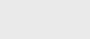2年前 (2021-10-03) |   抢沙发  159

[收起] 文章目录## streams流操作

### 过滤流

``````List<Book> javaBooks =
books.stream()
.filter(book -> book.getCategory().equals(JAVA))
.collect(Collectors.toList());``````

``````List<Book> lessThan42 =
books.stream()
.filter(book -> book.getPrice() < 42)
.collect(Collectors.toList());
``````

``````List<Book> priceLessThan42 =
books.stream()
.takeWhile(book -> book.getPrice() < 42)
.collect(Collectors.toList());
``````

`takeWhile`是在Java9中引入的。

``````List<Book> priceGreaterThan42 =
books.stream()
.dropWhile(book -> book.getPrice() < 42)
.collect(Collectors.toList());``````

### 查找流的不同元素

`distinct（）`方法通过应用`equals（）`方法返回由`distinct`元素组成的流。这是一个有状态的中间操作。

``````List<String> publisher =
books.stream()
.map(book -> book.getPublisher())
.distinct()
.collect(Collectors.toList());``````

### 有状态操作的问题

``````Set<Integer> seen = Collections
.synchronizedSet(new HashSet<>());

stream.parallel()
.map(e -> { if (seen.add(e)) return 0; else return e; })...
``````

### 截流

``````books.stream().limit(2)
.collect(Collectors.toList());
``````

``````books.stream().skip(2)
.collect(Collectors.toList());``````

### map

``````static List<String> mapToAuthors(List<Book> books) {
return books.stream()
.map(Book::getAuthor)
.collect(Collectors.toList());
}``````

### FlatMap

`flatMap`操作将一对多转换应用于流的元素，并将结果元素展平为新的流。

``````<R> Stream<R> flatMap(Function<? super T
, ? extends Stream<? extends R>> mapper);``````

`map`一样，传递给`flatMap`操作的`mapper`函数应该是无干扰和无状态的。

``````Set<String> s =
Stream.of(set1, set2)
.flatMap(Set::stream)
.collect(Collectors.toSet());
``````

`map``flatMap`操作之间的区别是–map仅应用变换。然而，`flatMap`也会使流变平。

``````static void flatMap() {

Arrays.asList(2, 3, 5, 7, 11, 13);

List<Integer> evenNumbers =
Arrays.asList(2, 4, 6, 8);

List<List<Integer>> evenOrPrime =

List<Integer> numbers =
evenOrPrime.stream()
.flatMap(Collection::stream)
.collect(Collectors.toList());

log.info("Flattened map : {}", numbers);
}``````

### 映射到原始流

``````static DoubleStream mapToPrice(List<Book> books) {
return books.stream()
.mapToDouble(Book::getPrice);
}
``````

``````static Stream<Double> box(DoubleStream doubleStream) {
return doubleStream.boxed();
}                                                                      ``````

### 流的匹配元素

Stream类提供了在流中查找匹配元素的方法。有两种方法。返回布尔值，例如anyMatch。另一个返回可选的，例如findAny。

### Any Match

``````static boolean hasAnyJavaBook(List<Book> books) {
return books.stream()
.anyMatch(book -> book.getCategory().equals(JAVA));
}
``````

### All Match

``````static boolean hasAllJavaBook(List<Book> books) {
return books.stream()
.allMatch(book -> book.getCategory().equals(JAVA));
}                                                                                 ``````

### None Match

``````static boolean hasNoJavaBook(List<Book> books) {
return books.stream()
.noneMatch(book -> book.getCategory().equals(JAVA));
}                                                                                  ``````

### 查找流的元素

#### Find Any

`findAny`方法返回描述流的任意元素的可选值，如果流为空，则返回空可选值。这是一种短路终端操作。此操作的行为是不确定的；它可以返回流的任何元素。

``````static Optional<Book> findAnyJavaBook(List<Book> books) {
return books.stream()
.filter(book -> book.getCategory().equals(JAVA))
.findAny();
}   ``````

Optional<T>类（java.util.Optional）是一个容器对象，用于表示值T的存在或不存在。如果findAny没有找到任何元素，那么它将返回空的Optional而不是null。

#### Find First

`findFirst`方法返回表示流的第一个元素的可选值，如果流为空，则返回空可选值。如果流没有遇到顺序，则可以返回任何元素。

``````static Optional<Book> findFirstJavaBook(List<Book> books) {
return books.stream()
.filter(book -> book.getCategory().equals(Category.JAVA))
.findFirst();
}    ``````

### 还原操作

#### Reduce

reduce方法T reduce（T identity，BinaryOperator<T>累加器）使用基于初始标识值的关联累加器函数（BinaryOperator:T，U->R）对流执行reduce操作。这是一个终端操作。

``````static double sum() {
List<Integer> numbers =
List.of(1, 2, 3, 4, 5);

return numbers.stream()
.reduce(0, (a, b) -> a + b);
}   ``````

``````private static double sum() {
List<Integer> numbers =
List.of(1, 2, 3, 4, 5);

return numbers.stream()
.reduce(0, Integer::sum);
}  ``````

### Min和Max

“减少”操作可用于查找最小值和最大值，如下所示：

``````List<Integer> numbers =
List.of(1,2,3,4,5);

Optional<Integer> min =
numbers.stream().reduce(Integer::min);

Optional<Integer> max =
numbers.stream().reduce(Integer::max);
``````

## 总结

• 您可以使用`filter``distinct``takeWhile``dropWhile``skip``limit`方法对流进行过滤和切片。
• 可以使用“`map`”和“`flatMap`”方法变换流的元素。`flatMap`操作也会使流变平。
• 您可以使用`findFirst``findAny`方法在流中查找元素。
• 可以通过对给定谓词使用`allMatch``anyMatch``anyMatch`方法来匹配流。
• 您可以使用`reduce`组合流的元素。### 注册